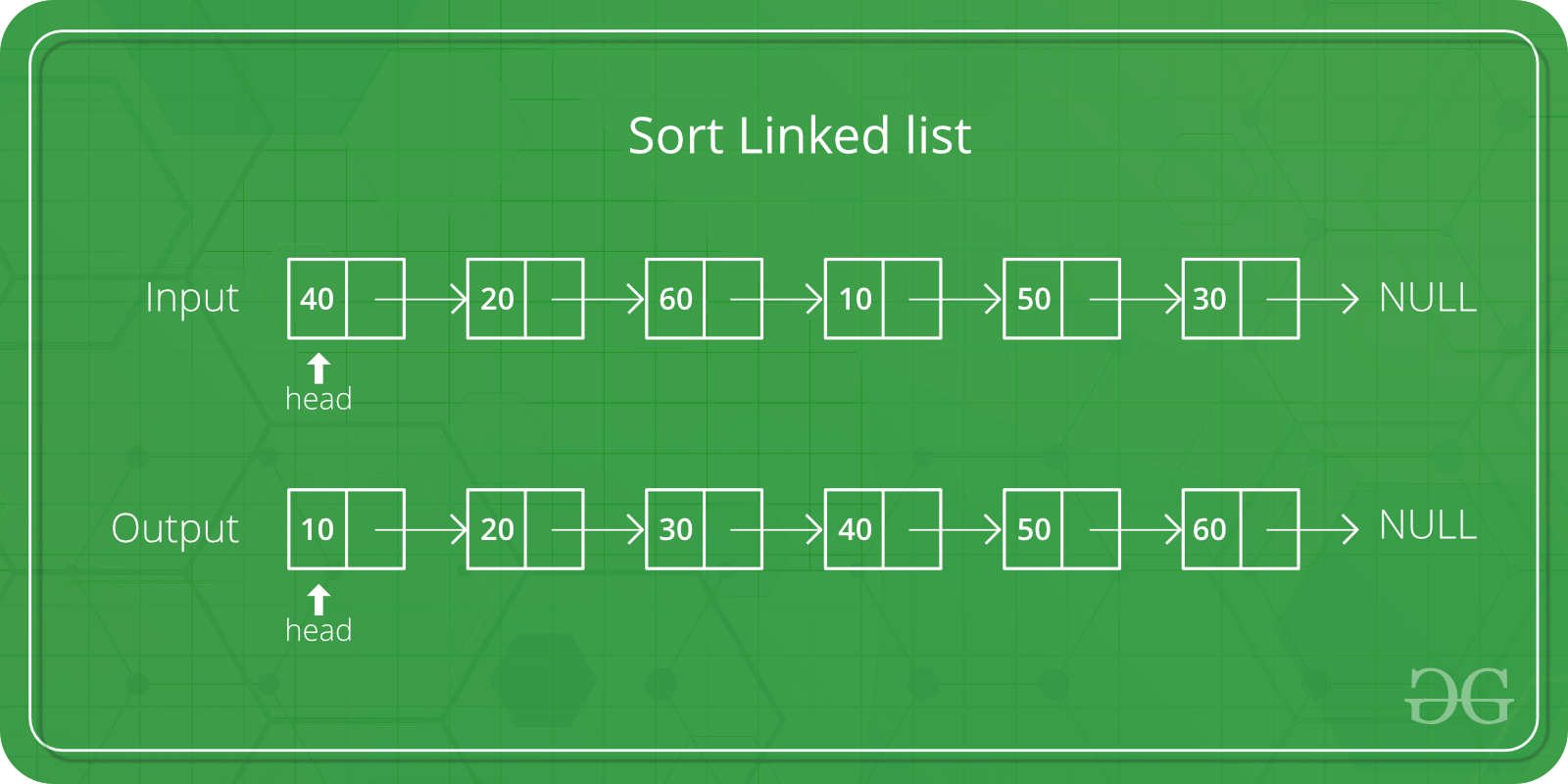GeeksforGeeks App
Open AppBrowser
Continue

# Java Program For Recursive Selection Sort For Singly Linked List – Swapping Node Links

Given a singly linked list containing n nodes. The problem is to sort the list using the recursive selection sort technique. The approach should be such that it involves swapping node links instead of swapping node data.Examples:

```Input: 10 -> 12 -> 8 -> 4 -> 6
Output: 4 -> 6 -> 8 -> 10 -> 12 ```

In Selection Sort, we first find the minimum element, swap it with the beginning node and recur for the remaining list. Below is the recursive implementation of these steps for the linked list.

```recurSelectionSort(head)
Initialize beforeMin = NULL

while ptr->next != NULL
if min->data > ptr->next->data
min = ptr->next
beforeMin = ptr
ptr = ptr->next

prevY->next = currX

Initialize temp = currY->next
currY->next = currX->next
currX->next  = temp    ```

The swapNodes(head_ref, currX, currY, prevY) is based on the approach discussed here but it has been modified accordingly for the implementation of this post.

## Java

 `// Java implementation of recursive``// selection sort for singly linked``// list | Swapping node links``class` `GFG{``    ` `// A Linked list node``static` `class` `Node``{``    ``int` `data;``    ``Node next;``};` `// Function to swap nodes 'currX'``// and 'currY' in a linked list``// without swapping data``static` `Node swapNodes(Node head_ref,``                      ``Node currX,``                      ``Node currY,``                      ``Node prevY)``{``    ``// Make 'currY' as new head``    ``head_ref = currY;` `    ``// Adjust links``    ``prevY.next = currX;` `    ``// Swap next pointers``    ``Node temp = currY.next;``    ``currY.next = currX.next;``    ``currX.next = temp;``    ``return` `head_ref;``}` `// function to sort the linked list using``// recursive selection sort technique``static` `Node recurSelectionSort(Node head)``{``    ``// If there is only a single node``    ``if` `(head.next == ``null``)``        ``return` `head;` `    ``// 'min' - pointer to store the node``    ``// having minimum data value``    ``Node min = head;` `    ``// 'beforeMin' - pointer to store``    ``// node previous to 'min' node``    ``Node beforeMin = ``null``;``    ``Node ptr;` `    ``// Traverse the list till the``    ``// last node``    ``for` `(ptr = head; ptr.next != ``null``;``         ``ptr = ptr.next)``    ``{``        ``// If true, then update 'min' and``        ``// 'beforeMin'``        ``if` `(ptr.next.data < min.data)``        ``{``            ``min = ptr.next;``            ``beforeMin = ptr;``        ``}``    ``}` `    ``// If 'min' and 'head' are not same,``    ``// swap the head node with the 'min' node``    ``if` `(min != head)``        ``head = swapNodes(head, head,``                         ``min, beforeMin);` `    ``// Recursively sort the remaining list``    ``head.next =``         ``recurSelectionSort(head.next);` `    ``return` `head;``}` `// Function to sort the given linked list``static` `Node sort(Node head_ref)``{``    ``// If list is empty``    ``if` `((head_ref) == ``null``)``        ``return` `null``;` `    ``// Sort the list using recursive``    ``// selection sort technique``    ``head_ref = recurSelectionSort(head_ref);``    ``return` `head_ref;``}` `// Function to insert a node at the``// beginning of the linked list``static` `Node push(Node head_ref,``                 ``int` `new_data)``{``    ``// Allocate node``    ``Node new_node = ``new` `Node();` `    ``// Put in the data``    ``new_node.data = new_data;` `    ``// Link the old list to the``    ``// new node``    ``new_node.next = (head_ref);` `    ``// Move the head to point to the``    ``// new node``    ``(head_ref) = new_node;``    ``return` `head_ref;``}` `// Function to print the linked list``static` `void` `printList( Node head)``{``    ``while` `(head != ``null``)``    ``{``        ``System.out.print(head.data + ``" "``);``        ``head = head.next;``    ``}``}` `// Driver code``public` `static` `void` `main(String args[])``{``    ``Node head = ``null``;` `    ``// Create linked list 10.12.8.4.6``    ``head = push(head, ``6``);``    ``head = push(head, ``4``);``    ``head = push(head, ``8``);``    ``head = push(head, ``12``);``    ``head = push(head, ``10``);` `    ``System.out.println(``    ``"Linked list before sorting:"``);``    ``printList(head);` `    ``// sort the linked list``    ``head = sort(head);` `    ``System.out.print(``    ``"Linked list after sorting:"``);``    ``printList(head);``}``}``// This code is contributed by Arnab Kundu`

Output:

```Linked list before sorting:
10 12 8 4 6